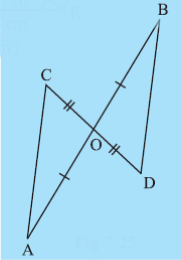Q

# In Fig 7.25,overline AB and overline CD bisect each other at O.State the three pairs of equal parts in two triangles AOC and BOD. Which of the following statements are true? bigtriangleup AOC cong bigtriangleup DOB

4. In Fig 7.25, and bisect each other at .

(i) State the three pairs of equal parts in two triangles and .

(ii) Which of the following statements are true?Views

i) The three pairs of equal parts in two triangles and  are:

CO = DO (given)

OA = OB (given )

( As opposite angles are equal when two lines intersect.)

ii) So by SAS congruency rule,

that is

Hence, option B is correct.

Exams
Articles
Questions